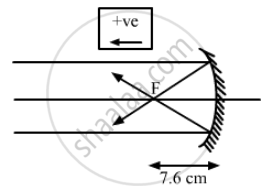Department of Pre-University Education, KarnatakaPUC Karnataka Science Class 12
Advertisement Remove all ads

# Find the Diameter of the Image of the Moon Formed by a Spherical Concave Mirror of Focal Length 7.6 M. the Diameter of the Moon is 3450 Km and the Distance Between the Earth - Physics

Sum

Find the diameter of the image of the moon formed by a spherical concave mirror of focal length 7.6 m. The diameter of the moon is 3450 km and the distance between the earth and the moon is 3.8 × 105 km.

Advertisement Remove all ads

#### Solution

Given,
Focal length of the concave mirror, f = − 7.6 m
Distance between earth and moon taken as object distance, u = −3.8 × 105 km
Diameter of moon = 3450 km
Using mirror equation:
$\Rightarrow \frac{1}{v} = \frac{1}{u} + \frac{1}{f}$
$\therefore \frac{1}{v} + \left( - \frac{1}{3 . 8 \times {10}^8} \right) = \left( - \frac{1}{7 . 6} \right)$
As we know, the distance of moon from earth is very large as compared to focal length it can be taken as ∞.
Therefore, image of the moon will be formed at focus, which is inverted.
$\Rightarrow \frac{1}{v} = - \frac{1}{7 . 6}$
⇒ v = −7.6 m

We know that magnification is given by:
$m = - \frac{v}{u} = \frac{d_{image}}{d_{object}}$
$\Rightarrow \frac{- ( - 7 . 6)}{( - 3 . 8 \times {10}^8 )} = \frac{d_{image}}{3450 \times {10}^3}$
$d_{image} = - \frac{3450 \times 7 . 6 \times {10}^3}{3 . 8 \times {10}^8}$ = $-$ 0.069 m = -6.9 cm
Hence, the required diameter of the image of moon is 6.9 cm.Concept: Concave Mirror
Is there an error in this question or solution?
Advertisement Remove all ads

#### APPEARS IN

HC Verma Class 11, 12 Concepts of Physics 1
Chapter 18 Geometrical Optics
Q 9 | Page 413
Advertisement Remove all ads

#### Video TutorialsVIEW ALL 

Advertisement Remove all ads
Share
Notifications

View all notifications

Forgot password?